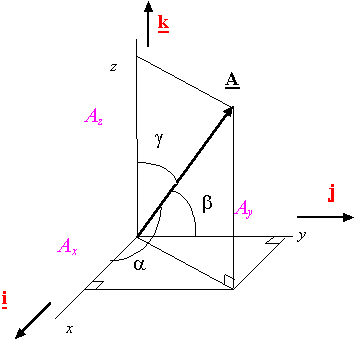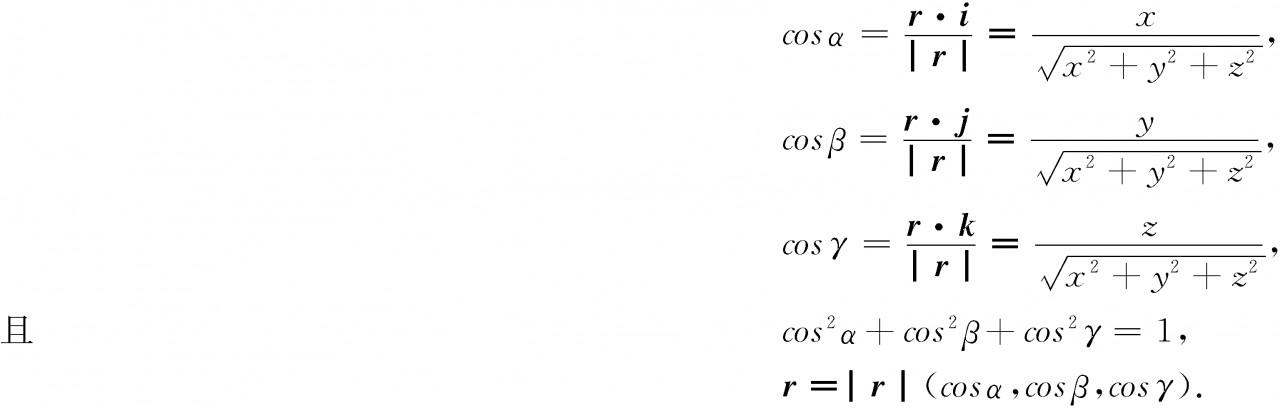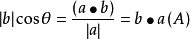• 姿态解算方向余弦四元数 ) 方向余弦 四元数 四元数是由实数和虚数组成的一组超复数，假设Q是一个四元数。 Q=w+ xi +yj +zj
姿态解算方向余弦矩阵基本概念已知欧拉角求方向余弦已知方向余弦求欧拉角四元数基本概念四元数表征姿态<1四元数的共轭<2四元数的逆四元数与方向余弦姿态表示的三种方法欧拉角方向余弦矩阵四元数$$spark-1$$
)
方向余弦矩阵
基本概念
方向余弦：
在坐标系R中设v为一个空间向量，在R坐标系下的投影为（vx,vy,vz）
与其x y z 轴分别成$\alpha \beta \gamma$角度，则$cos\alpha ,cos\beta,cos\gamma$分别为v在三轴的方向余弦，大小分别等于|vx|,|vy|,|vz|。
方向余弦矩阵（direction cosine matrix）
在一个平面内对向量进行旋转相当于对坐标进行旋转，初始状态下令载体坐标系和参考坐标系完全重合。
方向余弦矩阵便是存放着一系列方向余弦，设r(x,y,z)为参考坐标系，O(X1，Y1，Z1)为载体坐标系，载体转动时，载体坐标系会相对于参考坐标系转动，把载体坐标系的三个轴当做三个单位向量（vx,vy,vz）,每个载体坐标轴都可以在参考坐标系上找到三个对应的方向余弦。最终得到九个方向余弦，把九个方向余弦写成矩阵形式就是方向余弦矩阵。
而方向余弦矩阵的写法有两种，一种是载体坐标系相对于参考坐标系，一种是参考坐标系相对于载体坐标系。
$C_0^r=\begin{bmatrix}\\ c_{11} c_{12}c_{13} \\ c_{21} c_{22} c_{23} \\ c_{31}c_{32}c_{33} \end{bmatrix}或C_r^0=\begin{bmatrix}\\ c_{11} c_{21}c_{31} \\ c_{12} c_{22} c_{32} \\ c_{13}c_{23}c_{33} \end{bmatrix}$
C_0^r表称O系对于r系的方向余弦矩阵,这两个矩阵互为转置矩阵。
$C_{12}=cos(x_r.x_0)$载体坐标系上的\$x_0相对于参考坐标系上的x_r的方向余弦，
$C_{12}=cos(x_r,y_0)同理$ $y_0相对于参考坐标系上x_r的方向余弦$
已知欧拉角求方向余弦
$\psi \theta \gamma$
$\begin{bmatrix} cos \gamma cos\psi +sin\gamma sin\psi sin \theta& -cos\gamma sin\psi sin\theta & -sin\gamma cos\theta \\ sin\psi cos\theta&cos\psi cos\theta &sin\theta \\sin\gamma cos\psi -cos\gamma sin\psi sin\theta &-sin\gamma sin\psi -cos\gamma cos\psi sin\theta&cos\gamma cos\theta \end{bmatrix}$
已知方向余弦求欧拉角
方向余弦矩阵：$\begin{bmatrix} T_{11}&T_{21}&T_{31}\\T_{12}&T_{22}&T_{32} \\T_{13}&T_{23}&T_{33}\end{bmatrix}$
推出：
横滚角：$\theta=arcsinT_{32}$
俯仰角：
$\gamma=arctan(-\frac{T_{32}}{T_{33}})$
偏航角：
$\psi =arctan(\frac {T_{12}}{T_{22}})$
四元数
基本概念
四元数是由实数和虚数组成的一组超复数，假设Q是一个四元数。
                      Q =  w  +  xi +  yj +  zj

其中w表示四元数的实数大小，x,y,z表示虚数大小,实部单位为1，虚部单为为i,j,k。
四元数一般表示：
$\vec{q}=[w \vec{v}]=[w (xyz)]$
四元数表征姿态
其中w为实数部分，$\vec{v}$为矢量部分，首先讨论如何把一个空间向量转换到四元数。
假设在三维空间中有一个点（x,y,z),拓展到四元数空间有
$\vec{p}=[0(x y z)]$
$\vec{p}$就是三维空间中一点四元数的表示，而旋转是通过旋转四元数来实现的。现在假设空间中有一个向量$\vec{n}$,$(n_x,n_y,n_z)$,若用四元数来表示点p(x,y,z)绕向量$\vec{n}$旋转$\theta$角度后$p^,$
定义旋转四元数为$\vec{p}$则对于本次旋转有：
$\vec{p}=[cos$$(\frac{\theta}{2})$    sin$(\frac{\theta}{2})$$\vec{n}$]=[cos$(\frac{\theta}{2})$    $.$ sin$(\frac{\theta}{2})$ $\vec{n_x}$ $.$  sin$(\frac{\theta}{2})$$\vec{n_y}$ $.$   sin$(\frac{\theta}{2})$$\vec{n_z}$    ]
(其中.为分隔用)
其中

$\vec{n_x}$
$\vec{n_y}$
$\vec{n_z}$

$\vec{n_x}$=$n_x$*i
$\vec{n_y}$=$n_y$*j
$\vec{n_z}$=$n_z$*k

若想得到旋转后的矩阵只需要执行以下式子
$\vec{p^,}=\vec{q}\vec{p} \vec{q}^-1$
$(\vec{q}^-1为\vec{q}的逆矩阵)$
$想要推导下面的公式需要了解两个概念$
<1四元数的共轭
四元数的共轭就是让四元数的向量部分取负，记作
$\vec{p}$=[w  $\vec{v}$]=[w -$\vec{v}$]=[w (-x -y -z)]
四元数与他的共轭代表反向的角位移，因为相当于旋转轴反向
<2四元数的逆
四元数的逆就是他的共轭除以他的模
$\vec{q^-1} = \frac{(\vec{q}*)}{(|\vec{q}|)}$
一般使用单位四元数,所以他的逆和共轭是相等的
因为公式中涉及到四元数的乘法，在表示旋转时用叉乘，计算公式如下
$[w_1 (x_1 y_1 z_1)][w_2 ( x_2 y_2 z_2)]=\begin{bmatrix} w_1w_2 -x_1x_2 -y_1y_2-z_1z_2\\ \begin{pmatrix}w_1x_2 + x_1w_2 +z_1y_2-y_1z_2 \\w_1y_2+y_1w_2+x_1z_2-z_1x_2 \\w_1z_2+z_1w_2+y_1x_2-x_1y_2 \end{pmatrix} \end{bmatrix}$
example：
假设空间中有一个点p(0,1,0)饶z轴逆时针旋转90度，求旋转后的$p^,$点。理论上$p^,$点为（-1,0,0）。
首先把p(0,1,0)拓展成一个四元数$\vec{p}$=(0,0,j,0),然后定义旋转四元数，旋转轴为z轴化为单位向量（0,0,1）,因为旋转角度为90度所以------
$\vec{q}=(cos45^。,0,0,1*sin45^。k)=(\frac{\sqrt 2}{2},0,0,\frac{\sqrt 2}{2}k)$
单位四元数的逆的共轭是相等的
$\vec{q}^-1=(\frac{\sqrt 2}{2},0,0,-\frac{\sqrt 2}{2}k)$
由四元数叉乘计算公式 $\vec{p^,}=\vec{q}\vec{p} \vec{q}^-1$       用上述乘法公式计算
$\vec{q}\vec{p}=(0,\frac{\sqrt 2}{2}kj,\frac{\sqrt 2}{2}j,0)$
k*j=-i再乘以$\vec{q}^{-1}得到\vec{p}^{,}=[0,-i,0,0]$,带回去得到点[-1,0,0]即为旋转之后的坐标。
四元数与方向余弦
由前面的介绍知道了旋转四元数$q=cos\frac {\theta}{2}+x*sin\frac{\theta}{2}*i+y*sin\frac {\theta}{2}*j+z*sin\frac{\theta}{2}*k$
这里令$q_0=cos\frac{\theta}{2},q_1=sin\frac{\theta} {2},q_2=sin\frac{\theta}{2},q_3=sin\frac{\theta}{2},$
q=$q_0+q_1i+q_2j+q_3k$四元数旋转的方向余弦矩阵公式如下：
$\begin{bmatrix} x_b\\y_b\\z_b\end{bmatrix}=\begin{bmatrix}q_0^2+q_1^2-q_2^2-q_3^2& 2(q_1q_2+q_0q_3)&2(q_1q_3-q_0q_2) \\2（q_1q_2-q_0q_3）&q_0^2-q_1^2+q_2^2-q_3^2&2(q_0q_1+q_2q_3) \\2(q_1q_2-q_0q_3)&2(q_2q_3-q_0q_1)&q_0^2-q_1^2-q_2^2+q_3^2 \\ \end{bmatrix}\cdot\begin{bmatrix}x_n \\y_n \\z_n\end{bmatrix}$
$四元数表示的旋转矩阵公式$
姿态表示的三种方法
欧拉角
直观的欧拉角 横滚角roll  俯仰角pitch  偏航角 yaw
方向余弦矩阵
$\begin{bmatrix} cos \gamma cos\psi +sin\gamma sin\psi sin \theta& -cos\gamma sin\psi sin\theta & -sin\gamma cos\theta \\ sin\psi cos\theta&cos\psi cos\theta &sin\theta \\sin\gamma cos\psi -cos\gamma sin\psi sin\theta &-sin\gamma sin\psi -cos\gamma cos\psi sin\theta&cos\gamma cos\theta \end{bmatrix}$
四元数
$\begin{bmatrix} x_b\\y_b\\z_b\end{bmatrix}=\begin{bmatrix}q_0^2+q_1^2-q_2^2-q_3^2& 2(q_1q_2+q_0q_3)&2(q_1q_3-q_0q_2) \\2（q_1q_2-q_0q_3）&q_0^2-q_1^2+q_2^2-q_3^2&2(q_0q_1+q_2q_3) \\2(q_1q_2-q_0q_3)&2(q_2q_3-q_0q_1)&q_0^2-q_1^2-q_2^2+q_3^2 \\ \end{bmatrix}\cdot\begin{bmatrix}x_n \\y_n \\z_n\end{bmatrix}$
$spark-1$


展开全文• 个向量之间的方向余弦指的是这个向量之间的角度的余弦。 “方向余弦矩阵”是由两组不同的标准正交基的基底向量之间的方向余弦所形成的矩阵。方向余弦矩阵可以用来表达一组标准正交基与另一组标准正交基之间的...
前面在讨论两个不同坐标系之间的转换时都是通过欧拉旋转或者四元素来定义的。今天直接给出方向余弦阵的定义和用途。
方向余弦的定义
方向余弦是指在解析几何里，一个向量的三个方向余弦分别是这向量与三个坐标轴之间的角度的余弦。
如下图中，矢量A与坐标系三个轴$i,j,k$的夹角为$\alpha,\beta,\gamma$,则矢量A的方向余弦就是：
$[\cos(\alpha),\cos(\beta),\cos(\gamma)]$方向余弦矩阵
方向余弦矩阵是由两组不同的标准正交基的基底向量之间的方向余弦所形成的矩阵。方向余弦矩阵可以用来表达一组标准正交基与另一组标准正交基之间的关系。
使用$i_b,j_b,k_b$表示直角坐标系$ox_by_bz_b(b系)$的三个坐标轴的基向量，用$i_i,j_i,k_i$表示直角坐标系$ox_iy_iz_i(i系)$的三个坐标轴的基向量，则$i_b,j_b,k_b$（单位向量）分别可用$i_i,j_i,k_i$表示：
$\left\{\begin{matrix} i_b=(i_b\cdot i_i)i_i+(i_b\cdot j_i)j_i+(i_b\cdot k_i)k_i\\ j_b=(j_b\cdot i_i)i_i+(j_b\cdot j_i)j_i+(j_b\cdot k_i)k_i\\ k_b=(k_b\cdot i_i)i_i+(k_b\cdot j_i)j_i+(k_b\cdot k_i)k_i\\ \end{matrix}\right. \qquad(1)$
上式中，
$[(i_b\cdot i_i),(i_b\cdot j_i),(i_b\cdot k_i)]$为单位矢量$i_b$ 在坐标系$ox_iy_iz_i$的方向余弦；
$[(j_b\cdot i_i),(j_b\cdot j_i),(j_b\cdot k_i)]$为单位矢量$j_b$ 在坐标系$ox_iy_iz_i$的方向余弦；
$[(k_b\cdot i_i),(k_b\cdot j_i),(k_b\cdot k_i)]$为单位矢量$k_b$ 在坐标系$ox_iy_iz_i$ 的方向余弦。
将$i_b,j_b,k_b$分别在坐标系$ox_iy_iz_i$的方向余弦组成矩阵$M$(注意，按列组成)：
$M=\begin{bmatrix} i_b\cdot i_i &j_b\cdot i_i &k_b\cdot i_i\\ i_b\cdot j_i &j_b\cdot j_i &k_b\cdot j_i\\ i_b\cdot k_i &j_b\cdot k_i &k_b\cdot k_i \end{bmatrix} \qquad(2)$
则矩阵$M$即为b系到i系的坐标变换矩阵(推导过程略）。
假设点P在b系中的坐标为$\begin{bmatrix} x_b,y_b,z_b\end{bmatrix}^{T}$,在i系中的坐标为$\begin{bmatrix} x_i,y_i,z_i\end{bmatrix}^{T}$，则两者通过坐标旋转矩阵$M$联系：
$\begin{bmatrix} x_i\\y_i \\z_i \end{bmatrix}= M\cdot\begin{bmatrix} x_b \\y_b \\z_b \end{bmatrix} \qquad(3)$
方向余弦的应用
之前我们通过欧拉旋转或者四元素的方式得到两个坐标系之间的坐标变换矩阵$M$，而现在我们多了一种方法得到$M$。
假设上式中，$i$系为原始坐标系，$b$系为相机坐标系，那么我只要知道了相机坐标系（b系）的三个坐标轴的基向量$i_b,j_b,k_b$在$i$系中的坐标（方向余弦，式1），就可以得到坐标变换矩阵$M$（式2）。实际上只要知道两个基向量即可，剩下的用前两个基向量叉乘即可。


展开全文Cesium
• 方向余弦：用以确定向量的方向的量.向量(或有向直线)与坐标轴正向或基向量的交角称为向量的方向角.向量的方向角的余弦称为向量的方向余弦.一个向量的方向可以用它的方向角或方向余弦来确定.设向量r=xi+yj+zk的方向角...
方向余弦：用以确定向量的方向的量.向量(或有向直线)与坐标轴正向或基向量的交角称为向量的方向角.向量的方向角的余弦称为向量的方向余弦.一个向量的方向可以用它的方向角或方向余弦来确定.设向量r=xi+yj+zk的方向角为α，β，γ，则：向量夹角：向量之间夹角是将两个向量平移到共起点时所成的角。当两个向量同向时，夹角=0° 最小；当两个向量反向时，夹角=180° 最大。所以向量之间夹角范围是[0度, 180度]。

向量投影：设两个非零向量a与b的夹角为θ，则将|b|·cosθ 叫做向量b在向量a方向上的投影或称标投影（scalar projection），它是一个标量。其计算公式如下：其中a（A）是与向量a方向相同的单位向量。

附：函数的方向导数为梯度在该方向上的投影，说明如下：

f(x,y)在(x0,y0)沿方向(cosa,sina)的方向导数
f'(x0,y0)=f'x(x0,y0)cosa+f'y(x0,y0)sina
而在该点的梯度为
根据向量投影的定义
梯度f'x(x0,y0)i+f'y(x0,y0)j在方向(cosa,sina)的投影就是
f'x(x0,y0)cosa+f'y(x0,y0)sina
就等于在该点处的偏导数。

展开全文• 方向余弦矩阵(DCM)简介方向余弦矩阵(DCM)简介——定向运动学简介——1 前言这篇文章是翻译Starlino_DCM_... 这篇文章主要是介绍无人机方向余弦矩阵相关的知识，另外增加了定向运动学的主题。文章先通过一些理论...
方向余弦矩阵(DCM)简介方向余弦矩阵(DCM)简介——定向运动学简介——1   前言这篇文章是翻译Starlino_DCM_Tutorial.pdf而来，链接为：http://www.starlino.com/dcm_tutorial.html，各位看官可以对照着原文看，翻译不尽人意之处，请各位轻拍! 这篇文章主要是介绍无人机方向余弦矩阵相关的知识，另外增加了定向运动学的主题。文章先通过一些理论介绍，然后结合一些实际的例子展开讨论。该文章的算法是通过融合陀螺仪和加速度计数据，利用方向余弦矩阵(DCM、Direction Consine Matrix)的方法，以估计设备在空间中的方位。符号说明：向量以粗体文本标记 - 例如，“v”是向量，“v”是标量。2   DCM矩阵通常来说，定向运动学处理的问题为计算本体坐标系相对全局坐标系的相对方位。我们将本体坐标系定义为Oxyz，另外一个全局坐标系定义为OXYZ，两坐标系的具有相同的原点O，如图 1所示。本体坐标系的x、y、z轴对应的单位向量分别为i，j，k；全局坐标系X、Y、Z轴对应的单位向量分别为I，J，K。图1坐标系定义因此，通过定义，全局坐标系下的单位向量I，J，K表示形式可写为：IG = {1,0,0} T,      JG={0,1,0} T ,      KG = {0,0,1} T相应的，本体坐标系下的单位向量i，j，k表示形式可写为：iB = {1,0,0} T,      jB={0,1,0} T ,      kB = {0,0,1} T现在让我们看看是否可以在全局坐标系下表示向量i，j，k。让我们以向量i为例，写出它在全局坐标系下的坐标：iG = {ixG , iyG , izG} T作为示例，让我们分析X坐标轴的分量ixG，它的值为i向量投影到全局坐标系下X轴上的长度。ixG = |i| cos(X,i) = cos(I,i)其中，|i|为单位向量i的范数(长度)，cos(I,i)为向量I与i形成的夹角余弦，由于I与i均为单位向量，上式又可写成：ixG = cos(I,i) = |I||i| cos(I,i) = I.i其中，I.i为I与i的标量(点)乘积，为了计算标量积I.i。标量积在哪个坐标系中测量这些矢量并不重要,只要他们在同一个系统中表示，因为旋转不改变矢量的夹角。因此：I.i = IB.iB = IG.iG = cos(IB.iB) = cos(IG.iG) ，为了表示简便，本文一下部分将忽略I.i , J.j , K.k以及 cos(I,i), cos(J,j), cos(K,k)的上标。类似地，我们可以得出iyG = J.i , izG=K.i 。所以现在我们可以将全局坐标系中的向量i写为：iG= { I.i, J.i, K.i}T此外，类似的可以得出jG= { I.j, J.j, K.j} T , kG= { I.k, J.k, K.k} T现在本体坐标系下的 i, j, k在全局坐标系下有一套完整的表示，我们可以将这些向量组成一个方便的矩阵：Eq.1. 1       该矩阵为方向余弦矩阵，很明显，它是由本体坐标系和全局坐标系单位向量所有可能组合的角度的余弦组成。类似的，在本体坐标系中表示全局坐标系统的单位向量 IB, JB, KB本质上是对称的，并且可以简单的将I, J, K和 i, j, k交换实现，其结果是：IB= { I.i, I.j, I.k}T ,         JB= { J.i, J.j, J.k}T ,      KB= { K.i, K.j, K.k}T组成矩阵的形式为：Eq.1. 2现在很容易注意到DCMB = (DCMG)T或者DCMG = (DCMB)T，换句话说，两个矩阵是可以相互转换，我们将会在后面使用这一重要属性。另外，我们可以发现DCMB. DCMG = (DCMG)T .DCMG = DCMB. (DCMB)T = I3 ，其中I3是一个3*3的单位矩阵，换句话说DCM是正交的Eq.1. 3 其中， iGT. iG = | iG|| iG|cos(0) = 1    iGT. jG = 0DCM矩阵（通常也称为旋转矩阵）在定向运动学中具有很高的重要性，因为它定义了一个坐标系相对于另一个坐标系的旋转。如果我们知道任意向量在本体坐标系中的坐标，那么久可以利用DCM矩阵确定它在全局坐标系中的坐标，反之亦然。现在假设在本体坐标系中的向量rB= { rxB, ryB, rzB} T,让我们通过已知的旋转矩阵 DCMG确定其在全局坐标系中的坐标rG = { rxG , ryG , rzG } T。先从第一个坐标分量rxG开始，rxG = | rG| cos(IG,rG) ，根据定义，旋转是这样的变换，其不改变向量的尺度，并且不改变经过相同旋转的两个向量之间的角度，因此如果我们在不同的旋转坐标系中表示一些向量，则向量之间的范数和角度将不改变：| rG| = | rB| , | IG| = | IB| = 1 并且cos(IG,rG) = cos(IB,rB) 。根据这一属性可得出：rxG = | rG| cos(IG,rG) = | IB || rB| cos(IB,rB) = IB. rB = IB. { rxB, ryB, rzB} T上式代入IB= { I.i, I.j, I.k}T rxG = IB. rB = { I.i, I.j, I.k}T . { rxB, ryB, rzB} T = rxB I.i + ryB I.j + rzB I.k以相同的方式，可以表示出：ryG = rxB J.i + ryB J.j + rzB J.k rzG = rxB K.i + ryB K.j + rzB K.k最后，让我们以一个更紧凑的矩阵形式写：Eq.1. 4因此，DCM矩阵可以用于将在一个坐标系B中表示的任意向量rB转换为旋转的坐标系G。相同的逻辑，可以证明逆逻辑可以表示为：Eq.1. 5   最后：DCMB rG = DCMB DCMG rB = DCMGT DCMG rB = I3 rB = rB3   角速度到目前为止，我们已经有一个方法来定义一个坐标系相对于另一坐标系的方向旋转矩阵，DCM矩阵，它允许我们使用Eq.1.4和Eq.1.5容易地来回转换全局和本体坐标系。在本节中，我们将旋转矩阵作为时间的函数进行分析，这将有助于我们建立更新基于角速率的DCM矩阵的规则。假设任意一旋转向量r，并将其关于时间t的关系定义为 r(t)。现在考虑很小的时间间隔dt，并做出如下符号：r = r (t) , r’= r (t+dt) 和dr = r’ – r:图2  r(t)假设非常小的时间间隔内dt→0，矢量r以单位矢量u为旋转轴旋转了角度dθ到r’， u单位矢量垂直于由r和r’形成的平面，由于u为单位矢量，所以|u| = 1并且与 r x r’同向，我们可以通过如下推导得出：u = (r x r’) / |r x r’| = (r x r’) / (|r|| r’|sin(dθ)) = (r x r’) / (|r|2 sin(dθ))  Eq.2. 1因为旋转并不会改变一个矢量的长度，所以有| r’| = |r|。矢量r的线速度向量可以定义为：v = dr / dt = ( r’ – r) / dt       Eq.2. 2请注意，由于我们的dt→0因此向量r和dr之间的角度（我们称之为α）可以从由r，r’和dr构成的等腰三角形中找到。α = (π – dθ) / 2 ，由于 dθ→0，所以α→π/2，同时v⊥r。现在我们可以定义角速率矢量，表示角度θ 的变化速率以及旋转轴，定义如下：w = (dθ/dt ) u  Eq.2. 3将Eq.2.1代入上式可得：w = (dθ/dt ) u = (dθ/dt ) (r x r’) / (|r|2 sin(dθ))当dθ→0时，代入上式可得：w = (r x r’) / (|r|2 dt)  Eq.2. 4将r’ = r + dr代入上式，又由于dr/dt = v , r x r = 0 ，我们可推导出：w = (r x (r + dr)) / (|r|2 dt)= (r x r + r x dr)) / (|r|2 dt)= r x (dr/dt) / |r|2w =  r x v / |r|2­                Eq.2. 5上式建立了由已知线速度 v推导角速度的过程，通过矢量的叉乘特性，很容易推导出：v = w x r     Eq.2. 64   陀螺仪及角速度矢量三轴MEMS陀螺仪是一个能感应装置轴运动的装置，如果我们将该装置应用于本体坐标系中，然后分析一类全局坐标系(地球坐标系)的矢量，例如指向天的矢量K 或者指向北的矢量I，对于设备内的观察者来说，这些向量将围绕着设备中心旋转。假设 wx , wy , wz分别为轴 x, y , z的对应的陀螺仪的角速度速输出，单位为rad/s。如果我们以规则的小时间间隔dt查询陀螺仪，则陀螺仪输出告诉我们的是在该时间间隔期间，地球围绕陀螺仪的x轴旋转角度为dθx = wxdt，围绕y轴旋转角度为dθy = wydt并且关于z轴旋转角度 dθz = wzdt。这些旋转矢量可以用角速率矢量表征：wx = wx i = {wx , 0 , 0 }T , wy = wy j = { 0 , wy , 0}T , wz = wz k = { 0 , 0, wz }T其中， i,j,k 分别对应着本地坐标系各轴的方向向量。每个轴的旋转将会产生一定的线性位移，线性位移可表示为：dr1 = dt v1 = dt (wx x r) ;  dr2 = dt v2 = dt (wy x r) ;  dr3 = dt v3 = dt (wz x r)将三个线性位移相加可得：dr = dr1 + dr2 + dr3 = dt (wx x r + wy x r + wz x r) = dt (wx + wy + wz) x r有此产生的线速度可表示为：v=dr/dt=(wx + wy + wz) x r = w x r这里引入w = wx+wy+wz= {wx , wy , wz }上式类似于式Eq.2.6, 并且表明由角旋转矢量wx , wy , wz表征的轴x，y，z的三个小旋转的组合等效于由角旋转矢量w = wx + wy + wz = {wx , wy , wz }。请注意，我们强调这些都是小旋转，因为一般来说，当你结合大旋转，旋转的顺序执行变得重要，你不能简单地得出上述结论。我们的主要假设是，让我们通过使用Eq.2.6从线性位移到旋转的dt非常小，因此旋转dθ和线性位移dr也很小。实际上，这意味着陀螺仪查询间隔dt越大，我们的累积误差越大，我们稍后将处理这个误差。5   基于6DOF或9DOF IMU传感器的DCM滤波算法在本文的上下文中，6DOF设备是由3轴陀螺仪和3轴加速度计组成的IMU设备。9DOF设备是3轴陀螺仪，3轴加速度计和3轴磁力计的IMU设备。现在将基于右手定则的全局坐标系固定于地球，向量I指向北，向量 K指向天，向量J指向西。图3全局坐标系定义另外，定义连接到我们的IMU设备的本体坐标系如下：图4本体坐标系定义我们已经确立了陀螺仪可以测量角速度矢量的事实。让我们看看加速度计和磁力计的测量结果将如何进入我们的模型。加速度计是能感应重力的设备，重力矢量指向地心，与KB矢量相反。如果加速度计的三轴输出为A = {Ax , Ay , Az } ，假设无外力引起的加速度，并且误差已得到纠正，我们就可以确定KB= –A。磁强计类似于加速度计，可以感应北向磁场指向，犹如加速度计输出并不完美并且时常需要纠正和初始校准。如果纠正后的3轴磁场输出为M = {Mx , My , Mz }，根据我们的假设的模型 IB指向北，因此有 IB = M。确认IB和KB后便可计算出JB = KB x IB。因此根据加速度计和磁强计的输出便能给出DCM矩阵，表示为 DCMB或者DCMG。DCMG = DCMBT = [IB, JB, KB]T到目前为止，你可能会问自己，如果加速度计和磁力计在任何时间点能给出DCM矩阵，为什么我们需要陀螺仪？陀螺仪实际上是一个比加速度计和磁力计更精确的设备，它用于“微调”加速度计和磁力计返回的DCM矩阵。单靠陀螺仪并不能得到设备的方向，即不知道北在哪里和天的指向，但这些可以使用加速度计和磁力计确定，而是如果我们知道在时间t的设备的指向，表示为DCM矩阵DCM(t)，我们可以使用陀螺仪找到更精确的指向DCM(t + dt)。如果直接从加速度计和磁力计估计读数，其在形式上包含大量的噪声，包括外部（非重力）惯性力（即加速度）或不是由地球磁场引起的磁力。基于上述事实，需要寻求一种能够组合三种设备的输出数据的算法，用于最有效地估计设备在全局坐标系下的方位，下面我们将直接介绍该算法。将使用到的DCM如下所示：如果直接读取DCM的行，我们将得到向量 IB, JB, KB，我们将主要讨论向量KB (可以通过加速度计估计)和向量IB (可以通过磁力计估计)，向量JB 可由 JB = KB x IB简单计算获得。假设 t0时刻我们知道本体坐标系下指向天的矢量，记为 KB0，假设此时陀螺仪的输出我们也确定，记为 w = {wx , wy , wz }。经过一小段时间后，我们想知道指向天定矢量的位置，假设记为KB1G。通过Eq.2.6我们可以得出：KB1G ≈ KB0 + dt v = KB0 + dt (wg x KB0) = KB0 + ( dθg x KB0)其中， dθg = dt wg显然，估计KB的另外一种方法是通过加速度计输出计算，我们这里记为KB1A 。现实情况是，通过陀螺仪输出估计与通过加速度输出估计的值将会不一致。现在，我们可以用反向的方式，估计角速度 wa或角位移dθa ­=dt wa，通过加速度计的输出KB1A­ 以及Eq.2.5：w­a = KB0 x va / | KB0|2­其中va = (KB1A­ – KB0) / dt其中va = (KB1A­ – KB0) / dt, 几乎等于为KB0的线速度，并且| KB0|2­­ = 1。从而可得出：dθa ­= dt wa = KB0 x (KB1A­ – KB0)估计由KB1A和 KB1G组成的KB1方法的基本想法首先是将 dθ看作为dθa与 dθg的加权平均：dθ = (sa dθa + sg dθg) / (sa + sg­)，稍后将讨论权重，它们很快地被实验确定，以便实现期望的响应和噪声抑制。然后，对KB1的推导类似于如何计算KB1G：KB1 ≈ KB0 + ( dθ x KB0)同样， dθ 可以用来以相同的方式计算DCM矩阵的其他元素：IB1 ≈ IB0 + ( dθ x IB0)JB1 ≈ JB0 + ( dθ x JB0)三个向量彼此附接，并且均与由相同小的时间间隔 dt产生的角位移dθ叉乘转化而来。简而言之，这就是我们通过先前时刻 t­­0估计的矩阵DCM0，推导出 t1时刻的DCM1 算法。它是以相同的小时间间隔dt的递归应用，并且可以在任何时间递推出DCM矩阵。该DCM矩阵不会随着时间漂移太多，因为它被加速度计固定在指定的绝对位置；同时，不会由于外部加速度计太大噪声儿产生大漂移，因为DCM矩阵又使用了速率脱落的数据进行更新。到目前为止，我们并没有提到一个关于磁力计的词，其中一个原因是它并不是在所有的IMU单元中都有，上面的算法我们可以不使用它，但是该方法的结果指向会有一定的漂移，或许可以通过引入一个虚拟的指北磁力计，以保障模型的稳定性。下面将会讲如何集成磁力计的输出到我们的算法中，事实证明，过程非常的简单，因为磁力计类似于加速度计，唯一的区别是磁力计估计的不是天的指向 KB，而是估计指北向量 IB。遵循我们对加速度计所做的相同的逻辑，我们可以根据更新的磁力计读数确定角位移为：dθm ­= dt wm = IB0 x (IB1M­ – IB0)将上式并入加权平均公式可得：dθ = (sa dθa + sg dθg + sm dθm) / (sa + sg +­ sm)同样，可通过dθ 求得: DCM1­IB1 ≈ IB0 + (dθ x IB0)KB1 ≈ KB0 + (dθ x KB0)JB1 ≈ JB0 + (dθ x JB0)实际上，在将 KB1和IB1再次校正为垂直单位矢量之后，我们将计算JB1 = KB1 x IB1，注意，我们的所有逻辑都是近似的，并且取决于dt是否足够小，dt越大，误差越大。因此，如果向量IB0, JB0, KB0形成了有效的DCM矩阵，换句话说，它们彼此正交并且是单位向量，我们不能对关于 IB1, JB1, KB1的计算公式得出保证向量的正交性或长度，但是如果dt很小，我们不会得到太大的误差，我们需要做的是在每次迭代之后对它们进行校正化。首先，让我们看看如何确保两个向量再次正交。让我们考虑“几乎正交”的两个单位矢量a和b，换句话说，这两个矢量之间的角度接近90°，但不是精确的90°。我们要寻找一个与a正交并且在由向量a和b形成的平面中的向量b'，这一向量很容易找到，如图 5所示。首先通过叉乘的规则我们找到向量 c = a x b，c肯定垂直于a与b形成的平面。然后通过叉乘c与a得到b’ = c x a，因此，b’是我们正在寻找的与a正交的校正矢量，并且属于由a和b形成的平面。图5向量正交化通过三角定律的公式，我们可以推导出：b’ = c x a = (a x b) x a = –a (a.b) + b(a.a) = b – a (a.b) = b + d在上面的场景中，我们认为矢量a是固定的，并且我们推导出了与a正交的校正矢量b'。我们可以考虑相对称的问题——通过固定的矢量b，并找到经校正的向量a'。a’ = a – b (b.a) = a – b (a.b)第三种场景，我们想同时校正两个向量，我们认为它们都有同样的错误，所以直观的讲，上述两种场景的两个向量都应该用半校正。a’ = a – b (a.b) / 2b’ = b – a (a.b) / 2图6向量半正交化我们定义Err= (a.b)/2可得出a’ = a – Err * b    b’ = b – Err * a请注意，我们并不能证明a'和b'是正交的，但是我们提出了直观的推理，即如果我们应用上述校正变换，a'和b'之间的角度将接近90°。现在回到DCM矩阵的更新，我们在将DCM矩阵重新引入下一个循环之前应用以下纠正措施：Err = ( IB1 . JB1 ) / 2IB1’ = IB1 – Err * JB1 JB1’ = JB1 – Err * IB1 IB1’’ = Normalize[IB1’]JB1’’ = Normalize[JB1’]KB1’’ = IB1’’ x JB1’’其中，Normalize[a] = a / |a|因此，最后我们校正的矩阵DCM1 可以从IB1’’, JB1’’, KB1’’的组合得到。
展开全文• 方向余弦矩阵DCM&amp;amp;amp;amp;刚体的矢量—矩阵描述简介方向余弦矩阵刚体的矢量—矩阵描述 简介 我本意是为了学习理论力学，但是我对方向余弦矩阵的理解不够，所以不得不补习一下基础知识。这个过程里，我...线性代数 矩阵
• 姿态解算 四元数、方向余弦、欧拉角、Mahony滤波 说明：本文只是做了一些总结，需要一些对这方面的基础概念的了解。 一般人千万不要试图去深入探讨四元数 1. 方向余弦矩阵 方向余弦矩阵是使用欧拉角（pitch,roll,yaw...
• 说一句废话，概率密度函数的函数值是概率密度，表征该处概率变化快慢，而不是概率值。同时，概率密度函数不是概率函数，也不是分布函数。 个单位方向矢量夹角的余弦值求解过程：
• 设已知两点 M1(5,2,2),M2(4,0,3)M_1(5, \sqrt{2}, 2), M_2(4, 0, 3)M1​(5,2​,2),M2​(4,0,3) ，计算向量M1M2→\overrightarrow{M_1M_2}M1​M2​​ 模长 M1M2→=M2→−M1→=(−1,−2,1)∣M1M2→∣=(−1)2+(−2)2+...
• 四元数和欧拉角以及方向余弦的区别 2014-03-28 22:51 2078人阅读 评论(0) 收藏 举报  分类： 空间坐标变换的矩阵表示法（4）  版权声明：本文为博主原创文章，未经博主允许不得转载。 ...
• 方向余弦矩阵2.2.旋转矢量2.2.1等效旋转矢量2.2.2罗德里格参数2.3.四元数2.4 欧拉角3.相关转化 刚体定点旋转 1.定义 我们对Markdown编辑器进行了一些功能拓展与语法支持，除了标准的Markdown编辑器功能，我们增加了...矩阵
• 大概从去年冬天就开始接触姿态解算的内容了，中间走过很多弯路，当时一度迷糊在理论中，那时前辈让先学习方向余弦矩阵，但实际上，这个实际不常用。不过关于旋转矩阵的那个不正交矫正的原理倒是可以看看，非常巧妙。...经验分享 程序人生
• 前提：大地坐标系G系与大地固连，物体坐标系B系与飞机固连（X轴的方向为机头方向，Y轴的方向为左翼，Z轴方向朝天），初始状态下，令个坐标系完全重合。 问题：现在我在飞机上任取一点A，该在大地系的坐标为A0=...欧拉角 姿态结算 万向死锁 旋转矩阵
•  飞行器的姿态解算过程涉及到个坐标系，一个是运载体的机体坐标系，该坐标系与运载体固连，当运载体转动的时候，这个坐标系也跟着转动，我们假设运载体的坐标系为b系。另外一个是地理坐标系，即东北天坐标系，X轴...
• 该文章的算法是通过融合陀螺仪和加速度计数据，利用方向余弦矩阵(DCM、Direction Consine Matrix)的方法，以估计设备在空间中的方位。 符号说明： 向量以 粗体文本 标记 - 例如，“ v ”是向量，“v”是标量。 ...
• 按道理是应该根据求的切线的向量再做个向量的乘法然后才是法线的方向 18:00:45 为啥三个都是求切线呢 18:00:52 ？ 18:01:26 求切向不是切线吗 18:01:44 咋会是法线？ 18:02:20 但是以圆为例确实是对的 ...
• 但是位置是一个和原点有关的向量，其实位置是一个，把它和原点连起来，指向位置的向量就是位置向量。这种向量称之为有限向量。(Bound Vector) 而速度和力矩都和坐标系没有关系，他们自由存在于空间中，称之为自由...机器人学基础
• ## 余弦相似度

千次阅读 2017-01-10 14:51:13
余弦距离、欧氏距离和杰卡德相似性度量的对比分析 ...而要确定个向量方向是否一致，这就要用到余弦定理计算向量的夹角。 余弦定理描述了三角形中任何一个夹角和三个边的关系。给定三角形的三条边，可
• 篇文章我们讲了旋转矩阵和欧拉角，可知欧拉角是可以由旋转矩阵转化而来。 那么怎么从欧拉角转化为旋转矩阵呢？欧拉角（Euler angles）与旋转矩阵（Rotation Matrix）假设坐标系1的欧拉角yaw（Azimuth）、pitch...
• 余弦相似度，又称为余弦相似性，是通过计算个向量的夹角余弦值来评估他们的相似度。对于个向量，可以想象成空间中的条线段，都是从原点（[0, 0, ...]）出发，指向不同的方向条线段之间形成一个夹角：如果...
• 余弦相似度是通过测量个向量之间的夹角的余弦值来度量他们之间的一个相似度.0度角的余弦值是1,其他的任何角度的余弦值都不大于1,最小值是-1,从而个向量之间角度的余弦值确定了个向量是否指向同一个方向....
• 因工作需要比较个字符串的相似度比较，由于最短编辑距离算法不符合需求，就又找其他算法，在网上看到了另一个算法：余弦相似度算法。于是学习了一下，并写篇博客保存，以便学习以及日后用到。 代码如下: ...java
• 相比距离度量，余弦相似度更加注重个向量在方向上的差异，而非距离或长度上。 与欧几里德距离类似，基于余弦相似度的计算方法也是把用户的喜好作为n-维坐标系中的一个，通过连接这个与坐标系的原点构成一条...
• 前者是看成坐标系中两个 点 ，来计算两点之间的 距离 ； 后者是看成坐标系中两个 向量 ，来计算两向量之间的 夹角 。 前者因为是 点 ，所以一般指 位置 上的差别，即 距离 ； 后者因为是 向量 ，所以一般指 方向 ...
• （1）绘制余弦曲线和直线 /* 绘制余弦曲线和直线 在屏幕上显示0~360度的cos(x)曲线与直线f(x)=45*y+31的迭加图形。...图形迭加的关键是要在分别计算出同一行中个图形的列方向点坐标后，正确判断相互的...